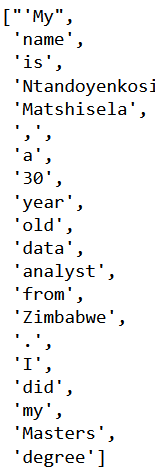top of page

# Introduction to Natural Language Processing (NLP)"Natural language processing is an area of research in computer science and artificial intelligence (AI) concerned with processing natural languages such as English or Mandarin. This processing generally involves translating natural language into data (numbers) that a computer can use to learn about the world. And this understanding of the world is sometimes used to generate natural language text that reflects that understanding."- Natural Language Processing in action, pg. 4.

The definition sets the scope of NLP in artificial intelligence, that we are analysing data, translating and drawing insights from the data.

We will import the following 2 packages 1. re and 2. nltk.tokenize. From nltk.tokenize will grab the word_tokenize and sent_tokenize packages

```# Libraries
import re
from nltk.tokenize import word_tokenize, sent_tokenize

```

We create an example of data we will use.

sample_text = "'My name is Ntandoyenkosi Matshisela, a 30 year old data analyst from Zimbabwe. I did my Masters degree at the National University of Science and Technology, Zimbabwe, where I specialised in Operations Research and Statistics, 2018. Prior to this I did a BSc in Operations Research and Statistics finishing it in 2015. My research interests are statistical computing and machine learning.😊 \n Most of the times, like 5 times a week, I tweet about #Python, #Rstats and #R. The tweeter handle is @matshisela😂. \n ◘Ŧ, ₦ ✔ \n I love gifts🎁, pizza 🍕, sandwich🥪'"

Search and Match

We can search or match for words and numbers. To do so we use re.search, for words we use “\w” and digits we “\d”. We can accommodate more letters and digits by adding a plus sign (+).

```# Let us match words
word_regex = r"\w+"
print(re.search(word_regex, sample_text))
number_regex = "\d+"
print(re.search(number_regex, sample_text))

```

Findall

We can search for capital letters by stating [A-Z] in our pattern. Further we include the full word which has the capital letter.

```# Write a pattern to match sentence endings: sentence_endings
capital_words = r"[A-Z]\w+"
print(re.findall(capital_words, sample_text))

```We can look for the lower case words by:

```lower_cases = r"[a-z]\w+"
print(re.findall(lower_cases, sample_text))

```Similarly digits can be found by:

```digits = r"\d+"
print(re.findall(digits, sample_text))

``````# Looking for words with hash tags
wild_card = r"[#]\w+"
print(re.findall(wild_card, sample_text))

```Word Tokenization

In the nltk library we can do the same things we have done above and indeed more. We download the following packages by running the following.

```import nltk

```

We can get the first 20 objects by:

```word_tokens = word_tokenize(sample_text)
word_tokens[:20]```Another cool thing is that we can split by sentences by:

```#sentences
sent_tokens = sent_tokenize(sample_text)
sent_tokens

```As you can see we have split the text file by sentences.

Another source of the data is tweeter where people post a lot of information mostly words. People also tag using @, for example @matshisela, and they use # to highlight a subject e.g. #Rstats. To delve into the analysis we use the following

```from nltk.tokenize import regexp_tokenize
from nltk.tokenize import TweetTokenizer
We can use the following pattern to filter the topics
pattern1 = r"#\w+"
hashtags = regexp_tokenize(sample_text, pattern1)
hashtags

```We can include the # hashtags and mentions in one line by

```pattern2 = r"[.@#]\w+"
mention_hashtags = regexp_tokenize(sample_text, pattern2)
mention_hashtags

```The TweetTokenizer can be instantiated by TweetTokenizer() which is used in the for loop.

```tknzr = TweetTokenizer()
all_tokens = [tknzr.tokenize(t) for t in sample_text]
all_tokens[:10]

```Emojis can be also used. The unicodes can be used to capture these like:

```## Non ascii tokenization
emoji = "['\U0001F300-\U0001F5FF'|'\U0001F600-\U0001F64F'|'\U0001F680-\U0001F6FF'|'\u2600-\u26FF\u2700-\u27BF']"
print(regexp_tokenize(sample_text, emoji))

```Charting Words

We can chart the distribution of the length of words. The following code outputs a chart that shows this distribution.

```import matplotlib.pyplot as plt
words = word_tokenize(sample_text)
word_lengths = [len(w) for w in words]
plt.hist(word_lengths)
plt.show()

```Word counts with bag of words

We can also show how many times a word has been used using the word tokenizer. We then get the most used word from the most used to the least used.

```## Word Counts with bag of words
from collections import Counter
# lets convert words to lower case so that we capture all words the same
tokens = word_tokenize(sample_text)
lower_tokens = [t.lower() for t in tokens]
word_number = Counter(lower_tokens)
print(word_number.most_common(10))

```## Simple Text Preprocessing

There are 3 preprocessing tasks one can do which are

1. Lemmatization

2. Lowercasing

3. Removing unwanted tokens

We can use the alphabetic words by using:

```# Let us look for alphabetic words
alpha_only = [t for t in lower_tokens if t.isalpha()]
alpha_only[:10]

```As you can see we have removed the commas and the non-alphabetic objects

We can remove the English stop words such as in this list to get a sense of the usage of the real words

```# Let us remove stop words, shall we
english_stops = ['the', 'they', 'i', 'my', 'to', 'and', 'a', 'in', 'is', 'did', 'of']
no_stops = [t for t in alpha_only if t not in english_stops]
no_stops[:10]

```We can then use the word net lemmatizer to find the most used words by:

```from nltk. stem import WordNetLemmatizer
wordnet_lemmatizer = WordNetLemmatizer()
lemmatized = [wordnet_lemmatizer.lemmatize(t) for t in no_stops]
# Bag of words
word_number2 = Counter(lemmatized)
print(word_number2.most_common((10)))

```As you can see that the most used words are research, which is what I am. Another striking use is Zimbabwe, where I come from too. Now think of, say if we have a large text file, more insights would be derived

The code to generate the above code and output is here

The concepts learnt came from the Datacamp NLP lesson